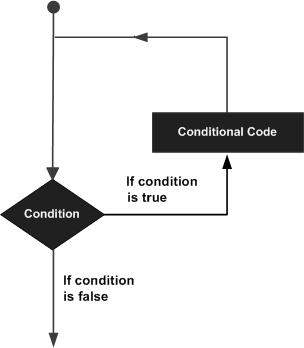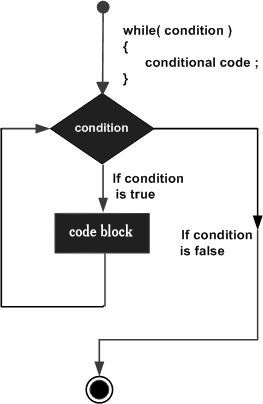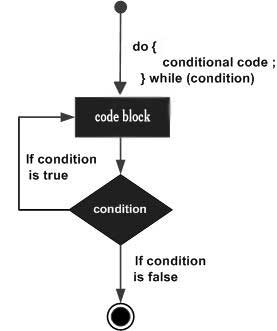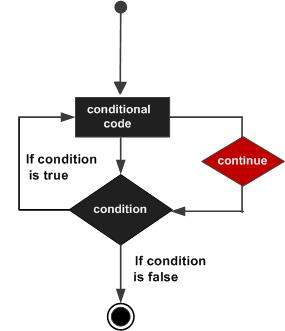# Computer Programming - Loops

Let's consider a situation when you want to print Hello, World! five times. Here is a simple C program to do the same −

```#include <stdio.h>

int main() {
printf( "Hello, World!\n");
printf( "Hello, World!\n");
printf( "Hello, World!\n");
printf( "Hello, World!\n");
printf( "Hello, World!\n");
}
```

When the above program is executed, it produces the following result −

```Hello, World!
Hello, World!
Hello, World!
Hello, World!
Hello, World!
```

It was simple, but again, let's consider another situation when you want to write Hello, World! a thousand times. We can certainly not write printf() statements a thousand times. Almost all the programming languages provide a concept called loop, which helps in executing one or more statements up to a desired number of times. All high-level programming languages provide various forms of loops, which can be used to execute one or more statements repeatedly.

Let's write the above C program with the help of a while loop and later, we will discuss how this loop works

```#include <stdio.h>

int main() {
int i = 0;

while ( i < 5 ) {
printf( "Hello, World!\n");
i = i + 1;
}
}
```

When the above program is executed, it produces the following result −

```Hello, World!
Hello, World!
Hello, World!
Hello, World!
Hello, World!
```

The above program makes use of a while loop, which is being used to execute a set of programming statements enclosed within {....}. Here, the computer first checks whether the given condition, i.e., variable "a" is less than 5 or not and if it finds the condition is true, then the loop body is entered to execute the given statements. Here, we have the following two statements in the loop body −

• First statement is printf() function, which prints Hello World!

• Second statement is i = i + 1, which is used to increase the value of variable i

After executing all the statements given in the loop body, the computer goes back to while( i < 5) and the given condition, (i < 5), is checked again, and the loop is executed again if the condition holds true. This process repeats till the given condition remains true which means variable "a" has a value less than 5.

To conclude, a loop statement allows us to execute a statement or group of statements multiple times. Given below is the general form of a loop statement in most of the programming languages −This tutorial has been designed to present programming's basic concepts to non-programmers, so let's discuss the two most important loops available in C programming language. Once you are clear about these two loops, then you can pick-up C programming tutorial or a reference book and check other loops available in C and the way they work.

## The while Loop

A while loop available in C Programming language has the following syntax −

```while ( condition ) {
/*....while loop body ....*/
}
```

The above code can be represented in the form of a flow diagram as shown below −The following important points are to be noted about a while loop −

• A while loop starts with a keyword while followed by a condition enclosed in ( ).

• Further to the while() statement, you will have the body of the loop enclosed in curly braces {...}.

• A while loop body can have one or more lines of source code to be executed repeatedly.

• If the body of a while loop has just one line, then its optional to use curly braces {...}.

• A while loop keeps executing its body till a given condition holds true. As soon as the condition becomes false, the while loop comes out and continues executing from the immediate next statement after the while loop body.

• A condition is usually a relational statement, which is evaluated to either true or false. A value equal to zero is treated as false and any non-zero value works like true.

## The do...while Loop

A while loop checks a given condition before it executes any statements given in the body part. C programming provides another form of loop, called do...while that allows to execute a loop body before checking a given condition. It has the following syntax −

```do {
/*....do...while loop body ....*/
}
while ( condition );
```

The above code can be represented in the form of a flow diagram as shown below −If you will write the above example using do...while loop, then Hello, World will produce the same result −

```#include <stdio.h>

int main() {
int i = 0;

do {
printf( "Hello, World!\n");
i = i + 1;
}
while ( i < 5 );
}
```

When the above program is executed, it produces the following result −

```Hello, World!
Hello, World!
Hello, World!
Hello, World!
Hello, World!
```

## The break statement

When the break statement is encountered inside a loop, the loop is immediately terminated and the program control resumes at the next statement following the loop. The syntax for a break statement in C is as follows −

```break;
```

A break statement can be represented in the form of a flow diagram as shown below −Following is a variant of the above program, but it will come out after printing Hello World! only three times −

```#include <stdio.h>

int main() {
int i = 0;
do {
printf( "Hello, World!\n");
i = i + 1;

if( i == 3 ) {
break;
}
}
while ( i < 5 );
}
```

When the above program is executed, it produces the following result −

```Hello, World!
Hello, World!
Hello, World!
```

## The continue statement

The continue statement in C programming language works somewhat like the break statement. Instead of forcing termination, continue forces the next iteration of the loop to take place, skipping any code in between. The syntax for a continue statement in C is as follows −

```continue;
```

A continue statement can be represented in the form of a flow diagram as shown below −Following is a variant of the above program, but it will skip printing when the variable has a value equal to 3 −

```#include <stdio.h>

int main() {
int i = 0;
do {
if( i == 3 ) {
i = i + 1;
continue;
}
printf( "Hello, World!\n");
i = i + 1;
}
while ( i < 5 );
}
```

When the above program is executed, it produces the following result −

```Hello, World!
Hello, World!
Hello, World!
Hello, World!
```

## Loops in Java

Following is the equivalent program written in Java that too supports while and do...while loops. The following program prints Hello, World! five times as we did in the case of C Programming −

You can try to execute the following program to see the output, which must be identical to the result generated by the above example.

```public class DemoJava {
public static void main(String []args) {
int i = 0;

while ( i < 5 ) {
System.out.println("Hello, World!");
i = i + 1;
}
}
}
```

The break and continue statements in Java programming work quite the same way as they work in C programming.

## Loops in Python

Following is the equivalent program written in Python. Python too supports while and do...while loops. The following program prints Hello, World! five times as we did in case of C Programming. Here you must note that Python does not make use of curly braces for the loop body, instead it simply identifies the body of the loop using indentation of the statements.

You can try to execute the following program to see the output. To show the difference, we have used one more print statement, which will be executed when the loop will be over.

```i = 0

while (i < 5):
print "Hello, World!"
i = i + 1
print "Loop ends"
```

When the above program is executed, it produces the following result −

```Hello, World!
Hello, World!
Hello, World!
Hello, World!
Hello, World!
Loop ends
```

The break and continue statements in Python work quite the same way as they do in C programming.